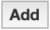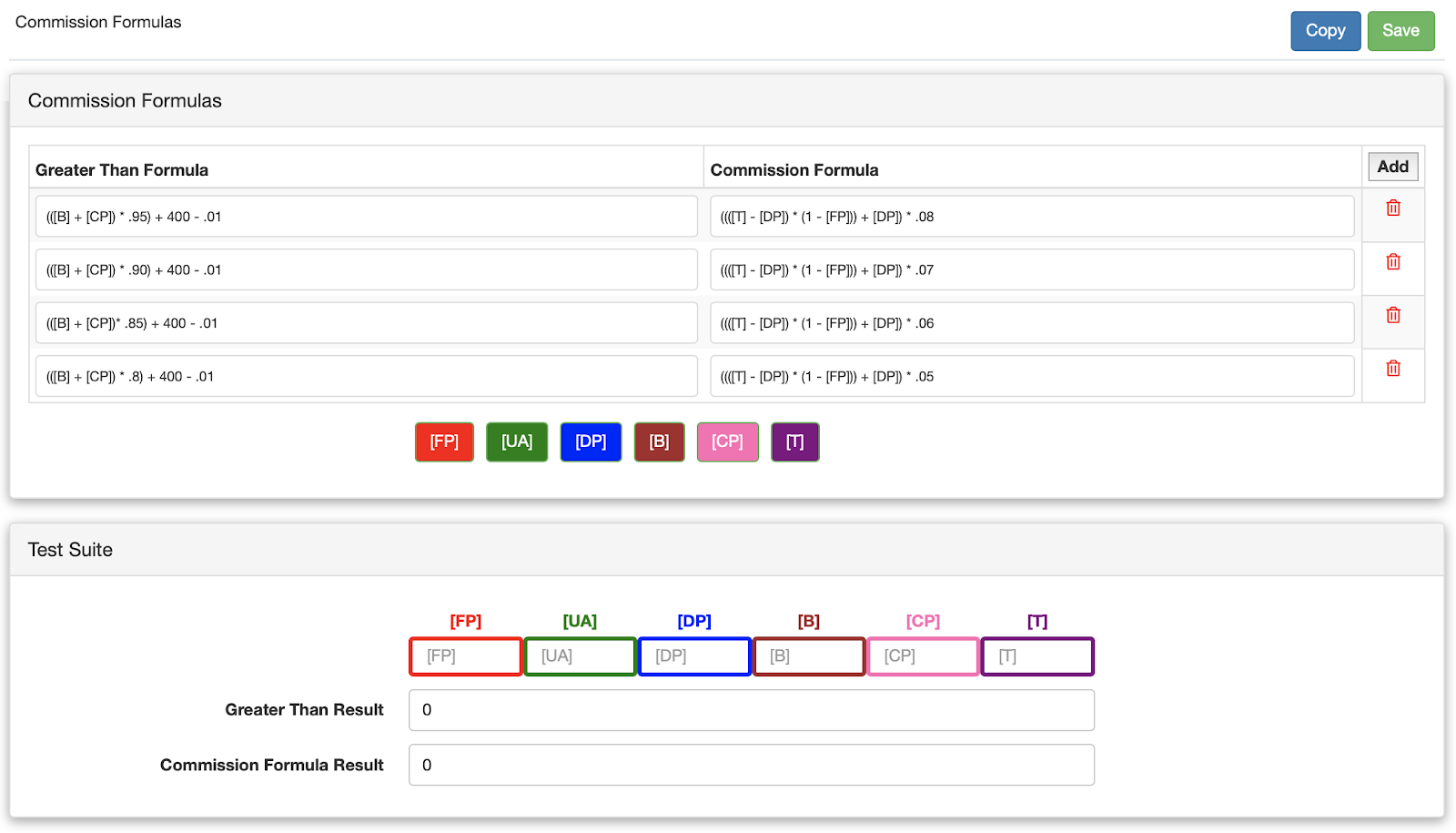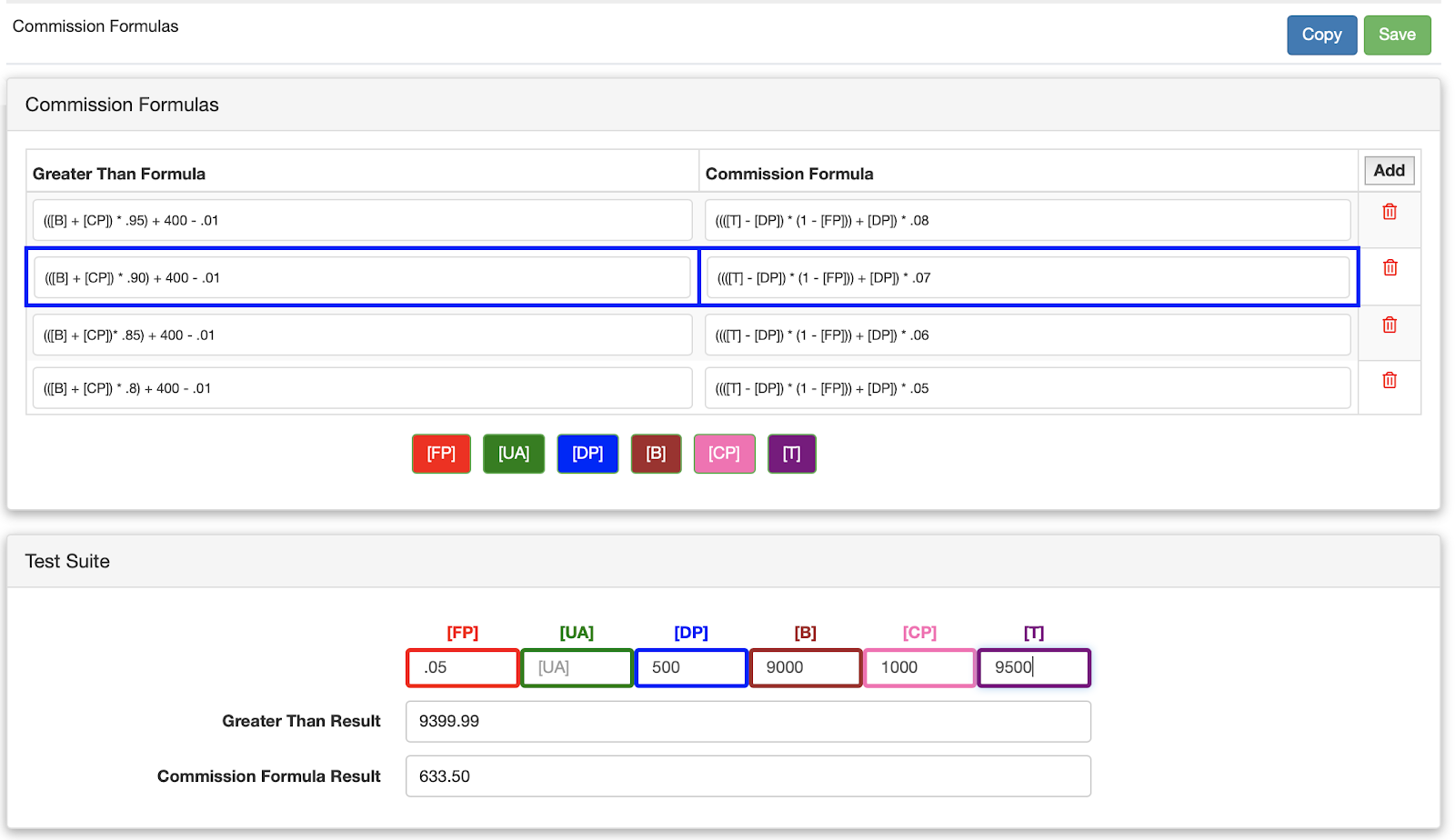# Commission Formulas

Use this section to tell the Leap app how to calculate commissions. Commission estimates will appear in the Results section if you have a field with Total Sale Amount and another field with Commission Estimate. Commission estimates are calculated first by checking if the Total Sale Amount entered by the user is greater than a specified formula. If the entered Total Sale Amount is greater than the Greater Than Formula then the resulting Commission Formula will be used to calculate the total commission earned. In the event the Total Sale Amount is greater than multiple formulas , Leap will use the highest calculated commission. You can add a new Commission Formula by clicking thebutton.## Placeholders

When creating formulas, Leap allows you to use placeholders that will tell the app to populate that field with data from inside of the app. The values and their representations are as follows:
[FP]
The Dealer Fee from the selected finance option in the estimates section of the Leap app. In the event no option is selected, 0 will be used.
[UA]
[DP]
The down payment that the user entered into the Leap app.
[B]
The total price of all added items, from all categories (except the “Custom Product” category). Note: The prices for these items come from the entered price you used when setting up the Price Guide.
[T]
The Total Sale Amount entered into the Results.
[CP]
The total price of all items from the Custom Product category.

## Example

Assume you want to calculate the commission on a sale at 7%. You want to remove any dealer fees from the finance options added to the estimate. You also want to make sure the total sale price is at least 90% of the total of all added items, plus \$400. Using the image above, assume the following:
• The total price of all added items is \$9,000 – [B]
• The total price of all added custom product items is \$1,000 – [CP]
• The total down payment entered is \$500 – [DP]
• The financing fee selected has a 5% dealer fee – [FP]
• The total sale amount entered is \$9,500 – [T]
In this example, the app would first evaluate the “greater than formula” to see the entered Total Sale Amount is greater than it’s result: (([B] + [CP]) * .90) + 400 – .01 (Note: Subtract .01 to simulate “Greater than OR equal to”) ((9000 + 1000) * .90) + 400 – .01 (10000 * .90) + 400 – .01 9000 + 400 – .01 9399.99 Since the total sale amount (9,500) is greater than 9399.99, this formula would be used. Now the app will evaluate the “Commission Formula” and use this price in the lead Results Commission Estimate field. (([T] – [DP]) * (1 – [FP])) + [DP]) * .07 ((9500500) * (1 – .05)) + 500) * .07 ((9000 * .95) + 500) * .07 (8550 + 500) * .07 9050 * .07 \$633.50 – Total commission

## Test Suite

You can use the test suite to test your Commission Formulas. It will show you which formula is being used by highlighting it in blue and display the Commission Formula Result at the bottom of the page.